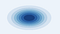# Sampling From a Gaussian (Box-Muller method)

In the previous post, we discussed how to sample from a distribution when the CDF has a closed-form solution and can be inverted. In this post, we will look into sampling from a gaussian.

Consider the scenario of sampling from a standard normal distribution. Using the inverse sampling method involves computing the CDF of the function and its inverse. Let's consider the CDF of a standard normal distribution:

As evident from the above formula, we cannot obtain a closed-form formula for the CDF due to the integral involved.

The Box-Muller method is genius trick to overcome this by producing two independent standard normals from two independent uniforms.

To understand the magic behind the box-muller method, let's look at the following integral:

This cannot be calculated directly. Instead, we calculate the square of the integral. The following derivation will depict why it is a good idea to do so.

Substituting x = rcos(θ) and y = rsin(θ),

The Box-Muller algorithm is a probabilistic interpretation of the trick shown above. Consider a function f, which is the product of two independent standard Gaussians.

Since the distribution is radially symmetric we can convert x and y to polar coordinates.Contour plot for 2-D Gaussian. From the radial symmetry, we can deduce θ is uniformly distributed.

Given the radial symmetry, we can say that θ is uniformly distributed in [0, 2π]

The CDF can now be written as:

Now that we have a closed-form formula for the CDF, we can use inversion sampling.

Solving the above equation and putting all the pieces together we have:

We now have a methodology to generate samples X and Y from two independent Gaussians by sampling from two uniform distributions.

The sampling method described above involves computing the trigonometric quantities sine and cosine, which can be expensive. Instead, one alternative is to sample points from a unit square and then rejecting the samples that lie outside the unit circle. For more details refer to the “Polar Form” section in this article.

In the next post, we will discuss a more generalized algorithm for sampling.

# Reference

1. https://www.math.nyu.edu/faculty/goodman/teaching/MonteCarlo2005/notes/GaussianSampling.pdf

## More from Mohammed Suhail

Website: mohammedsuhail.net